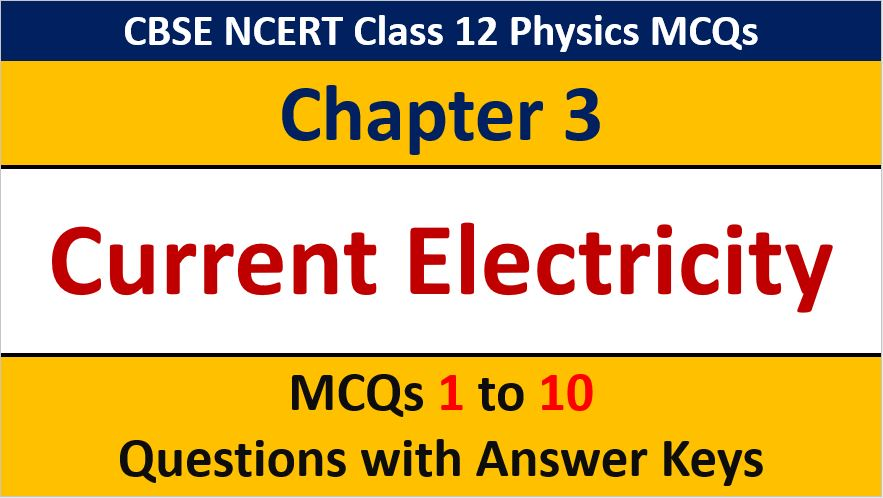## MCQ Questions for Class 12 Physics Chapter 3 Current Electricity with Answer Keys

If you are preparing for CBSE Class 12 Board Exam Term 1 which will be an objective type questions paper, then you need to practice multiple choice questions of Class 12 Physics Chapterwise. In this article of AKVTutorials, you will get MCQ Questions for Class 12 Physics Chapter 3 Current Electricity with Answer Keys.

MCQ Questions for Class 12 Physics Chapter 3 Question No 1:

Which of the following is responsible for the flow of current in a conductor?

Option A : Protons

Option B : Free electrons

Option C : Positive ions

Option D : Protons and holes

Option B : Free electrons

MCQ Questions for Class 12 Physics Chapter 3 Question No 2:

The current in a conductor varies with time t as 1=2t + 3t2 ,where I is in ampere and t in seconds, Electric charge flowing through a section of the conductor during t=2 sec to t=3 sec is

Option A : 10 C

Option B : 24 C

Option C : 33 C

Option D : 44 C

Option B : 24C

MCQ Questions for Class 12 Physics Chapter 3 Question No 3:

How much work is required to move a 6 μC charges from the negative to the positive terminal of a 9 V battery?

Option A : 54×10-3 J

Option B : 54×10-9 J

Option C : 54×10-6 J

Option D : 54×10-12 J

Option C : 54×10-6 J

MCQ Questions for Class 12 Physics Chapter 3 Question No 4:

The resistance of a conductor is

Option A : Inversely proportional to the length

Option B : Directly proportional the square of the radius

Option C : Inversely proportional to the square of the radius

Option D : Directly proportional to the square root of the length

Option C : Inversely proportional to the square of the radius

MCQ Questions for Class 12 Physics Chapter 3 Question No 5:

What is the unit for specific resistivity?

Option A : ohm cm2

Option B : ohm cm-1

Option C : ohm cm

Option D : ohm cm-2

Option C : ohm cm

MCQ Questions for Class 12 Physics Chapter 3 Question No 6:

The physical quantity having the dimensions [M-1L-3T3A2] is

Option A : Resistance

Option B : Resistivity

Option C : Electrical conductivity

Option D : Electromotive force

Option C : Electrical conductivity

MCQ Questions for Class 12 Physics Chapter 3 Question No 7:

What length of the wire (specific resistance 48 × 10-8 Ωm)is needed to make resistance of 4.2 mm)

Option A : 1.1 m

Option B : 3.1 m

Option C : 2.1 m

Option D : 4.1 m

Option A : 1.1 m

MCQ Questions for Class 12 Physics Chapter 3 Question No 8:

The dimensions of a block are 1 cm × 1 cm × 100 cm. If the specific resistance  of the material is 3 × 10-7  Ωm, then the resistance between two opposite rectangular bases is

Option A : 3 × 10-9 Ω

Option B : 3 × 10-7 Ω

Option C : 3 × 10-5 Ω

Option D : 3 × 10-1 Ω

Option B : 3 × 10-7 Ω

MCQ Questions for Class 12 Physics Chapter 3 Question No 9:

The ratio of masses of three wires is 1 : 2 : 3 and that of their lengths is 3 : 2 : 1. If the wires are made of same material, the ratio of their resistances will be

Option A : 1 : 1 : 1

Option B : 1 : 2 : 3

Option C : 9 : 4 : 1

Option D : 27 : 6 : 1

Option D : 27 : 6 : 1

MCQ Questions for Class 12 Physics Chapter 3 Question No 10:

Two wires of the same material have lengths l and 2l and areas of cross-section 4 A and A respectively. The ratio of their specific resistances would be

Option A : 1 : 2

Option B : 8 : 1

Option C : 1 : 8

Option D : 1 : 1

Option D : 1 : 1

CBSE Class 12 Physics Chapterwise MCQ
1 : Electric Charge & Fields MCQs
| 1 to 10 | 11 to 20
2 : Electrostatics Potential and Capacitance MCQs
| 1 to 10 | 11 to 20
3 : Current Electricity MCQs
| 1 to 10 | 11 to 20
4 : Moving charges and Magnetism MCQs
| 1 to 10 | 11 to 20
5 : Magnetism and Matter MCQs
| 1 to 10 | 11 to 20
6 : Electromagnetic Induction MCQs
| 1 to 10 | 11 to 20
7 : Alternating Current MCQs
| 1 to 10 | 11 to 20
8 : Electromagnetic Waves MCQs
| 1 to 10 | 11 to 20
9 : Ray Optics and Optical Instruments MCQs
| 1 to 10 | 11 to 20
10 : Wave Optics MCQs
| 1 to 10 | 11 to 20
11 : Dual Nature of Radiation MCQs
| 1 to 10 | 11 to 20
12 : Atoms MCQs
| 1 to 10 | 11 to 20
13 : Nuclei MCQs
| 1 to 10 | 11 to 20
14 : Semi Conductor Electronics MCQs
| 1 to 10 | 11 to 20
15 : Communication Systems MCQs
| 1 to 10 | 11 to 20